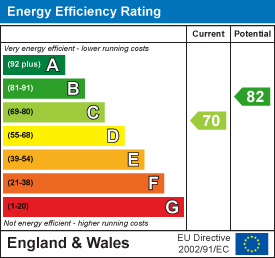•£1,950.00 To Let
•£1,950.00 To Let
•£1,950.00 To Let
•£1,950.00 To Let
•£1,950.00 To Let
•£1,950.00 To Let
•£1,950.00 To Let
•£1,950.00 To Let
•£1,950.00 To Let
•£1,950.00 To Let
•£1,950.00 To Let
•£1,950.00 To Let
•£1,950.00 To Let
•£1,950.00 To Let
•£1,950.00 To Let
•£1,950.00 To Let
•£1,950.00 To Let
•£1,950.00 To Let
•£1,950.00 To Let
•£1,950.00 To Let
•£1,950.00 To Let
•£1,950.00 To Let
•£1,950.00 To Let
•£1,950.00 To Let